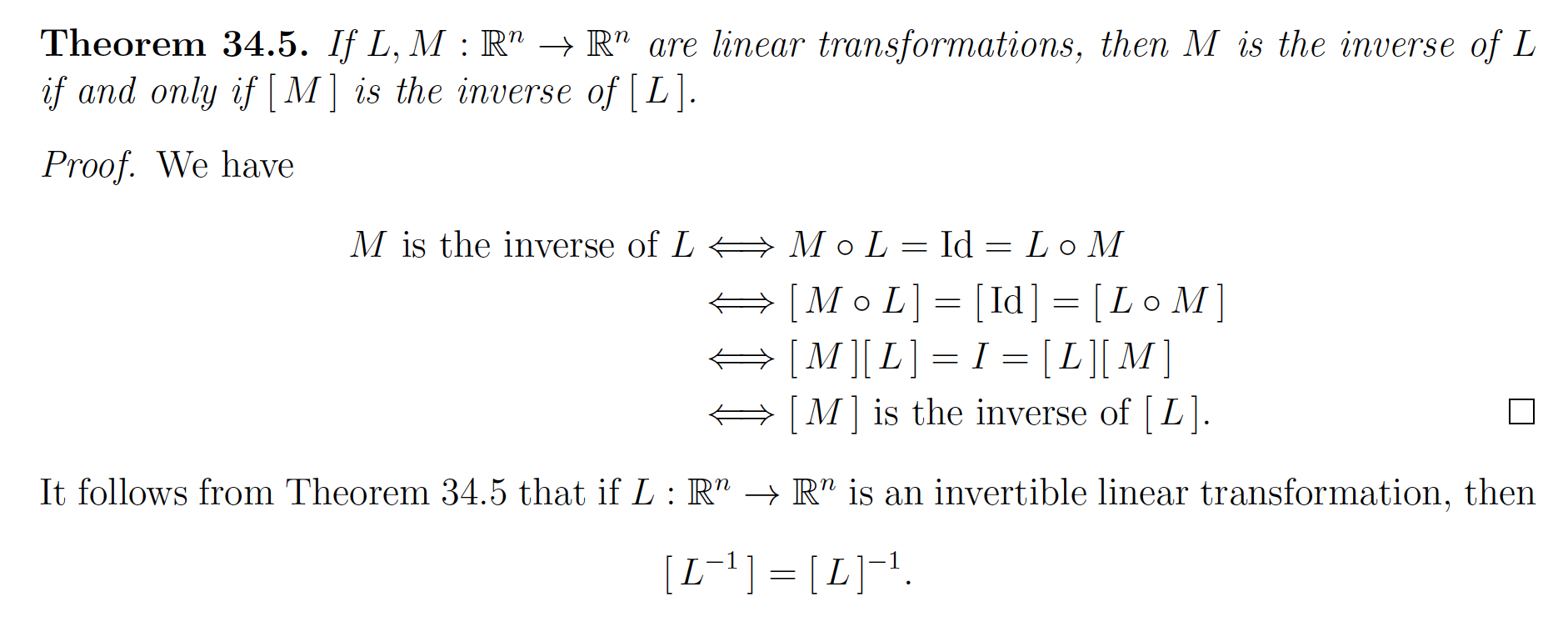# Linear Transformation §

This has extremely important applications for Robotics and Virtual Reality. Used for Rigid-Body Transformations

Notes from MATH115, Articulated Robotics, and Steven M. Lavalle’s VR lectures.

Think of linear transformations as just functions in higher dimensions, i.e. . We often represent them as a Matrix Transformation. For example, a 2D Linear Transformation:

### Linear Transformation §

A function is called a linear transformation if for every , and for every we have

Now, what’s super cool is that we can represent a linear transformation as a Matrix Transformation!

If is a linear transformation, then is a Matrix Transformation with corresponding matrix , i.e. for every . The matrix is given by

Proof is at page 201 of the pdf.

##### Some Properties §
1. 0 always maps to 0, i.e.
2. Linear Transformations are always an Odd Function, i.e.
3. You can do Composition of Function on Linear Transformation, where
• This is super useful, for example, when you apply multiple rotations, see Rotation Matrix

### Inverse Linear Transformations §

This is actually more important than I thought. For example, if you apply a rotation linear transformation, how do you undo that rotation?

• Answer: Represent linear transformation as Matrix Transformation, and get the inverse of the matrix. Proof at page 215if is an invertible linear transformation, then

### Kernel and Range of Linear Transformations §

Given a linear transformation , there are two important sets that carry with them information about .

Kernel Definition: Let be a (linear) transformation. The kernel of is Note that . It is a subspace of it.

Range Definition: Let be a (linear) transformation. The range of is

Note that . It is a subspace of it.

Theorem 35.6. Let be a linear transformation with standard matrix . Then

### One-to-One and Onto Linear Transformations §

Wow, as I revisit these notes, This is Serendipity with the stuff I learned in SE212 about what Functions are.

If is a linear transformation, then is one-to-one if and only if .

Let be a linear transformation. Then L is one-to-one if and only if .

is a one-to-one correspondence if and only if is invertible.